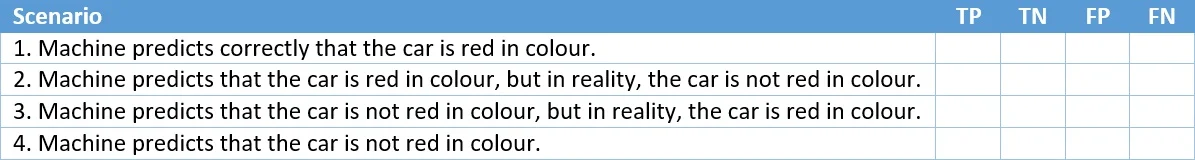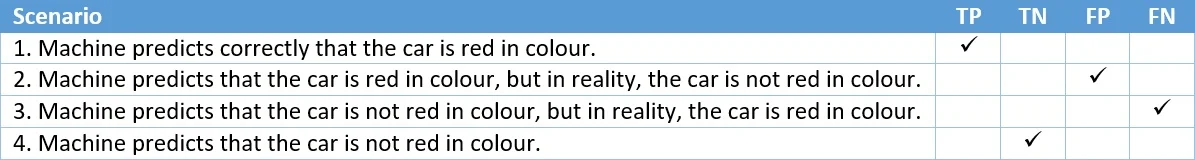# vs.eyeandcontacts.com

## Unit 7: Evaluation

Worksheet 1:
Question 1: Why is the process of evaluation important in any AI model?
When we are at the modelling stage, we take up various modelling types for a project. The selection of the model we take up depends on how we are going to evaluate the project. An efficient evaluation model proves helpful in selecting the most suitable modelling method that would represent our data. Therefore, the model evaluation stage is an essential part of the process of selecting an appropriate model for a project.

Question 2: Fill in the correct terms in the table given below.Worksheet 2:
Consider an AI prediction model that predicts the colour of a car in each image provided to it. No

Check the correct classification with respect to the given scenario:Worksheet 3:
Question 1: Is high accuracy equivalent to good performance?
Let us take the example of forest fire. Assuming that the model always predicts that there is no fire. But in reality, there is a 2% chance of forest fire breaking out. In this case, for 98 cases, the model will be right but for those 2 cases in which there was a forest fire, then too the model predicted no fire.
Here,
True Positives = 0
True Negatives = 98
Total cases = 100

Therefore, accuracy becomes: (98 + 0) / 100 = 98%

98% is a fairly high accuracy for an AI model. But this parameter is useless for us as the actual cases where the fire broke out are not taken into account. Hence, there is a need to look at another parameter which takes account of such cases as well.

Question 2: What percentage of accuracy is reasonable for representing a good performance?
No amount of percentage is reasonable for representing a good performance. Even though the accuracy is 98% or 99%, it is useless. Let us take the example of forest fire. Assuming that the model always predicts that there is no fire. But in reality, there is a 2% chance of forest fire breaking out. In this case, for 98 cases, the model will be right but for those 2 cases in which there was a forest fire, then too the model predicted no fire.
Here,
True Positives = 0
True Negatives = 98
Total cases = 100

Therefore, accuracy becomes: (98 + 0) / 100 = 98%

98% is a fairly high accuracy for an AI model. But this parameter is useless for us as the actual cases where the fire broke out are not taken into account.

Question 3: Is good precision equivalent to good model performance? Why?
Precision is equivalent to good model performance in few cases but not in all cases. Taking the example of forest fire, in this case, assuming that the model always predict that there is a forest fire irrespective of the reality. In this case, all the Positive conditions would be taken into account that is, True Positive (Prediction = Yes and Reality = Yes) and False Positive (Prediction = Yes and Reality = No). In this case, the firefighters will check for the fire all the time to see if the alarm was True or False. Similarly, here if the Precision is low (which means there are more False alarms than the actual ones) then the firefighters would get complacent and might not go and check every time considering it could be a false alarm. This makes Precision an important evaluation criteria. If Precision is high, this means the True Positive cases are more, giving lesser False alarms.

Question 4: What is the difference between Precision and Recall?

Precision: Precision is defined as the percentage of true positive cases versus all the cases where the prediction is true.
Precision = TP/(TP + FP) x 100%
Precision takes into account True Positive (TP) and False Positive (FP).

Recall: Recall can be defined as the fraction of positive cases that are correctly identified.
Recall = TP/(TP + FN)
Recalls considers True Positive (TP) and False Negative (FN).